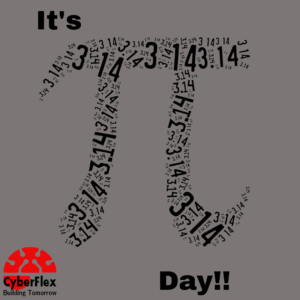# Pi π Day – Appreciating Mathematics for Machine LearningAs today is PI day, it seems to be a good idea to celebrate how mathematics has helped define machine learning.

Mathematical knowledge is vital to understanding and applying machine learning algorithms. From understanding uncertainty and intervals of prediction; to choosing parameter setting with a validation strategy, mathematical concepts help to implement machine learning.

An advanced level of mathematics experience is necessary. A deep dive into machine learning often requires a multi-dimensional effort, and a series of mathematical formulations can work to advance the theory and efficiency of machine learning.